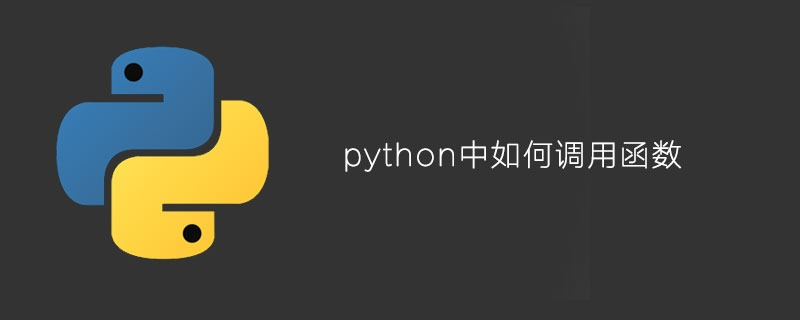## python中如何调用函数

470次阅读1.定义函数–封装独立的功能

2.调用函数–享受封装的成果

```# 定义一个函数，声明2个形参
def my_max(x, y) :
# 定义一个变量z，该变量等于x、y中较大的值
z = x if x > y else y
# 返回变量z的值
return z
# 定义一个函数，声明一个形参
def say_hi(name) :
print("===正在执行say_hi()函数===")
return name + "，您好！"
a = 6
b = 9
# 调用my_max()函数，将函数返回值赋值给result变量
result = my_max(a , b) # ①
print("result:", result)
# 调用say_hi()函数，直接输出函数的返回值
print(say_hi("孙悟空")) # ②```

```result: 9
===正在执行say_hi()函数===

```def my_max(x, y) :
# 返回一个表达式
return x if x > y else y```